Mathematics

# Absolute Value Inequalities

1.6k views

28 September 2020

## Introduction

There are different aspects of the absolute value function and one is the interesting concept of absolute value inequalities, which is the intersection of absolute value and inequalities.

Absolute value inequalities deal with the inequalities ... ... However, there exist several techniques in solving absolute value inequalities like using basic properties, considering the cases, graph visualization, etc. .. Let us explore and thus conceptualize the steps involved in solving absolute value inequalities.

## Absolute Value Inequalities

An absolute value equation has no solution if the absolute value expression equals a negative number since an absolute value can not be negative.

So, we can write as…..absolute value inequality as a compound inequality.

Absolute value inequalities deal with the inequalities $$( < , ≤ , > , ≥ )$$ on the expressions with absolute value sign.

 Example:

Solve the absolute value inequality :- $$x: |x-9|<4$$

Solution

The simple and easy way to solve the equation:-

\begin{align} & \left| x-a \right|=\{~-x\text{ }+\text{ }a \qquad \text{for}~x\text{ }<\text{ }a \\\\ & \{~~x\text{ }-\text{ }a\qquad \text{for}~x~\ge a \\ \end{align}

For any real number x

x, its absolute value is defined as

 $$\left| x \right|\text{ }=\text{ }\left\{ \text{ }x \text{ if }x\text{ }>\text{ }0\quad 0 \text{ if }x\text{ }=\text{ }0\quad-x \text{ if }x\text{ }<\text{ }0~ \right\}$$

The function $$f (x) = ∣x∣$$ is also called the modulus function

What are some basic rules which one must be aware of when solving absolute value inequalities? Let us move onto such properties of absolute value inequalities.

### Basic Properties of Absolute value inequalities

Let $$x$$ be a flexible or an algebraic expression and allow a be the real number such that $$a > 0.$$

And then while solving absolute value inequalities, keep in mind the following:

• $$∣x∣ ≤ a ⇔ − a ≤ x ≤ a$$
• $$∣x∣ ≥ a ⇔ x ≤ − a \text{ or } x ≥ a$$
• $$∣x∣ < a ⇔ −a < x < a$$
• $$∣x∣ > a ⇔ x < −a \text{ or } x > a$$

And for f(x) and g(x), functions of x, so we have these inferring absolute value inequalities:

• $$∣f (x) ∣ ≤ g (x) ⇔ −g (x) ≤ f(x) ≤ g(x)$$
• $$∣f (x) ∣ ≤ g (x) ⇔ −g (x) ≤ f(x) ≤ g(x) \text{ or } f (x) ≥ g(x)$$
• $$∣f (x) ∣ < g (x) ⇔ −g (x) < f(x) < g(x)$$
• $$∣f (x) ∣ > g (x) ⇔ f (x) < −g (x) \text{ or } f (x) > g (x)$$

Here are some other properties of absolute value inequality:

1. $$∣a + b∣ ≤ ∣ a ∣ + ∣b∣$$ with equality if both have the similar sign, i.e. $$ab > 0$$
2. $$∣ a − b ∣ ≤ ∣a∣ + ∣b∣$$ with equality if they have different signs, i.e. $$ab < 0.$$

## How to solve absolute value inequalities

Here, we learn how to solve absolute value inequalities:

$$| x |$$ value is the distance of x from zero.

For instance, both $$–2$$ and $$+ 2$$ are two units from zero, as you can see in the image below: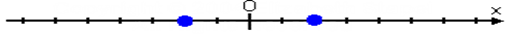This means their absolute values will both be $$2;$$ that is, we have:

$|–2| = |+2| = 2$

Let us look at some absolute value inequalities problems for better understanding of how to solve absolute value inequalities.

### Absolute Value Inequalities Problems

 Example:

Solving inequalities with absolute value $$|x| < 3,$$ and how to graph absolute value inequalities.

Solution

In this absolute value inequality, what is being asked is solving absolute value inequalities and to find all the x-values that are less than three units away from zero in either direction, so the solution is going to be the set of all the points that are less than three units away from zero.

First, I'll draw a number line: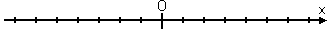Looking at the inequality, I see that number 1 will work as a solution, as well as –1 because each of these is less than three units from zero. The number 2 will work, as well as –2. But 4 will not work, and neither will –4 because they are too far away from zero. Even 3 and –3 won't work (though they're right on the edge), because this is a "less than" (but not equal to) inequality.

Still, the number 2.99 will work, as well as –2.99. In other words, all the points between –3 and 3, but not actually including –3 or 3, will work as solutions to this inequality. So, graphing the absolute value inequality, the solution looks like this: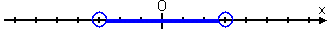Translating this picture into algebraic symbols, I get the following solution:

$–3 < x < 3$

 Example:

Solving inequalities with absolute value: $$| 2x + 3 | < 6.$$

Solution

Since this is the "smaller than" absolute value inequality, my first step is to clear the absolute value according to the "less than" pattern.

\begin{align} &| 2x + 3 | < 6\\\\&–6 < 2x + 3 < 6\end{align}

This is the pattern for "less than". Continuing, I'll subtract 3 from all three "sides" of the inequality:

\begin{align}&–6 – 3 < 2x + 3 – 3 < 6 – 3\\&–9 < 2x < 3\\&−\frac{9}{2} < x < 3/2\end{align}

The solution to the actual absolute value inequality, $$|2x + 3| < 6$$, is this interval:

 $$− \frac{9}{2} < x < \frac{3}{2}$$

## Graphing Absolute Value Inequalities

One more way of solving absolute value inequalities is by graphing absolute value inequalities. Now, how to graph absolute value inequalities?

### Graphing Absolute Value Inequalities

 Example:

$$Y ≥ 2 |x+1| −5$$

Solution

The steps involved in graphing absolute value inequalities are pretty much the same as for linear inequalities.

Step 1 Look at the inequality symbol to see if the graph is dashed

Step 2 Draw the graph as if it were an equality.

Step 3 Pick a point not on the line to test to see where to shade.

So, we first note that

≥ is used so the absolute value graph will have a solid line. Next, we move onto graphing the absolute value inequalities. This equation has three components to look at.

First is the

+1 inside the absolute value. Then will shift our graph to the left by one unit.

Next is the

−5 outside. This will shift our graph down by five units.

Last, there's a

2 multiplying the absolute value term. This will explain what slope the lines will have. Applying the all to the basic absolute value graph, so we get: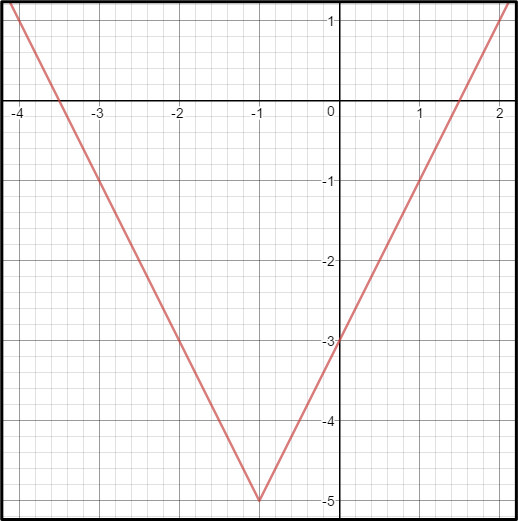$Y = 2 |x+1| −5$

The final step is to pick a point not on the line to test. Since (0,0) is not on the line, I will choose that.

\begin{align}&(0) ≥ 2 | (0) + 1 | - 5\\\\&0 ≥ 2 |1 | - 5\\\\&0 ≥ 2 - 5\\\\&0 ≥ - 3\end{align}

So is zero greater than or equal to negative three? Accordingly, ( 0,0 ) is inside the graph, we shade the inside portion.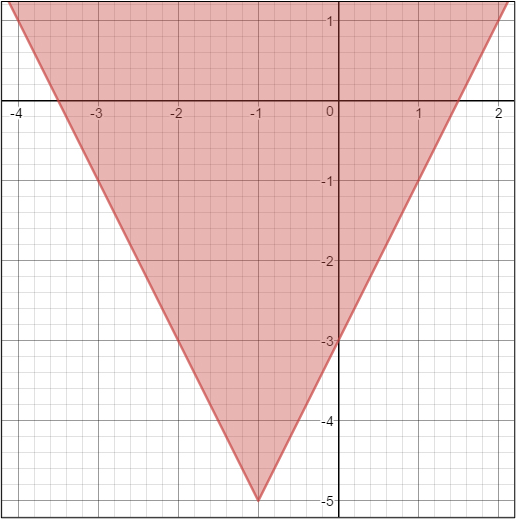$$Y ≥ 2 |x+1| −5$$

## Absolute Value Inequalities Worksheet

Cuemath has many absolute value inequalities worksheets which are made carefully such that they will help in solidifying concepts of absolute value inequalities and how to solve absolute value inequalities. These absolute value equations and inequalities worksheet are available on this link- https://www.cuemath.com/algebra/inequalities-involving-absolute-values/

## Summary

Absolute value inequalities will make 2 solution sets due to the nature of absolute value. We solve by writing two equations: one equal to a positive value and one equal to a negative value.

Absolute value inequality solutions can be verified by graphically.

The concept of absolute value is tricky because equations with absolute value generally have more than one solution. This chapter helps to alleviate the difficulties of absolute value equations and inequalities and understand how to solve absolute value inequalities by providing concrete steps to follow when solving absolute value inequalities. It also introduces the idea of a critical point. This idea, as well as the steps that we use, will be useful in other topics of algebra--such as graphing absolute value inequalities in more than one variable. Thus, it is important to master them now.

Written by Neha Tyagi

## What do absolute values with inequalities mean?

An absolute value inequality is an inequality that has an absolute value sign with a variable inside.

## What are the rules of absolute value?

In mathematics, the important rule of the absolute value of a real number x, denotes | x |, is the non-negative value of x without regard to its sign. In other words, |x| = x if x is positive, and |x| = −x if x is negative (in which case −x is positive), and |0| = 0.

## What is the purpose of absolute value?

When you see an absolute value in a problem or equation, it means that whatever is inside the absolute value is always positive. Absolute values are generally used in problems involving distance and are sometimes used with inequalities.

## Why is an absolute value always positive?

No. Absolute value is always positive. Since this is the distance a number is from 0, it would always be positive.

## Is 0 an absolute value?

The absolute value of 0 is 0. (The reason is why because we don't say that the absolute value of a number is positive: Zero is neither negative nor positive.)

GIVE YOUR CHILD THE CUEMATH EDGE
Access Personalised Math learning through interactive worksheets, gamified concepts and grade-wise courses